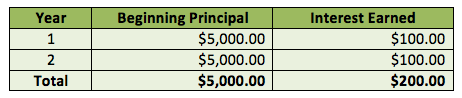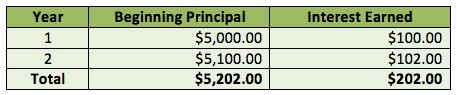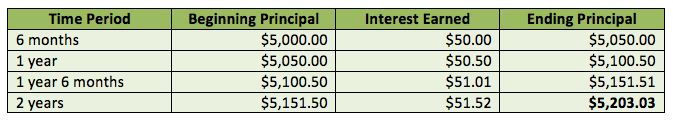# Simple vs. Compound Interest: Let’s Break It DownHere’s a riddle: two people invest the same amount of money in GICs that pay the exact same interest rate. But one earns more than the other. How can this be?

The answer is in the interest, itself, and the fact that it was calculated differently in the respective cases.

When you purchase a GIC, two kinds of interest can be paid: simple and compound. With simple interest, the bank only pays you interest based on the initial principal. The interest is calculated as:

Principal x Interest Rate x Amount of Time

So if you invested \$1,000 in a 1-year GIC at 1.95% that paid simple interest, you would earn:

\$1,000 x 1.95% x 1 Year = \$19.50

And it would be paid out to you at the end of your term, typically in an account outside of the GIC structure (so it might be deposited into your savings account).

With compound interest, by contrast, every time interest is earned on your GIC, it’s added to your principal. This way, the next time the bank pays you interest, it does so based on the now-larger principal. In other words, you’re now earning interest on top of interest, not just interest on the principal.

The calculation is essentially the same, except that it’s performed multiple times throughout the time you hold your investment.

Let’s take a look at how simple and compound interest work using an example. Say you can invest \$5,000 in a 2-year GIC paying 2.00% interest per year. How much will you earn if you go either the simple or compound interest routes?

Simple InterestCompound InterestAs you can see, you’ll earn more with GICs that pay compound interest because your principal grows with each interest payment. Mind you, it also means that you have to wait until the end of the GICs term to receive any money.

So far we’ve distinguished between simple and compound interest. But in the example above, in both cases interest is paid annually. What if interest is paid at a greater interval, such as semi-annually?

Nothing changes for the simple interest calculation, other than how often you’re paid. But the compound interest calculation is different.

Again, let’s assume you invest \$5,000 in a GIC paying 2.00% interest over 2 years. With a semi-annual payment, this means that half (i.e. 1.00%) of the interest is paid every 6 months. Here’s how the final numbers would look:Because of the more frequent interest payments, you would earn slightly (just slightly!) more interest when it is paid semi-annually.

To sum things up, compound interest can be very powerful over time. If you don’t need the money, consider leaving interest payments in your GIC until the product matures. You’ll gain interest on interest and have more money at the end of the day.Flickr: Dave Dugdale